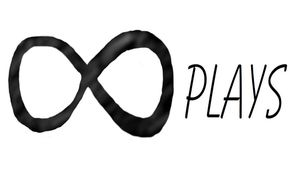By Bob

I was experimenting with different ways to make a lithophane and tried to combine intersect with surface, and the results were pretty good for making a flat image on a curved surface which isn't ideal for a lithophane but makes some cool designs, here is the code:

``````/*[Surface Cylinder]*/
Path_to_PNG_File="/home/none3/Desktop/path.png";
Show_Intersect_Plane = false;
Hollow = true;
Depth = -.25;   //[-1:.01:0]
Cylinder_size= 51; //[10:.01:200]
Cylinder_height = 50;//[1:.01:200]
Cylinder_shape = 100; //[3:1:200]
Rotate_x=0; //[0:.01:360]
Rotate_y=0; //[0:.01:360]
Rotate_z=0; //[0:.01:360]
Move_x = 0; //[-200:.01:200]
Move_y = 0; //[-200:.01:200]
Move_z = 0; //[-200:.01:200]
scale_x = -0.05; //[-2:.01:-0.01]
scale_y = -0.05; //[-2:.01:-0.01]
scale_z = 1.5; //[-10:.01:10]

module cutplane(){
difference(){
cylinder(h=Cylinder_height,r1=Cylinder_size/2,r2=Cylinder_size/2,\$fn=Cylinder_shape,center=true);
Intersect();
}}

module Intersect(){
intersection(){
rotate([90,180,0])
rotate([Rotate_x,Rotate_y,Rotate_z])
translate([-Move_x,-Move_z,-Move_y])
scale([scale_x,scale_y,scale_z])
translate([Cylinder_size/2,Cylinder_size/2,-Cylinder_size/2])
surface(file=Path_to_PNG_File,center=true,invert =false,convexity=10);
}
cylinder(h=Cylinder_height+1,r1=Cylinder_size/2.1,r2=Cylinder_size/2.1,\$fn=Cylinder_shape,center=true);
}

module InsideTube(){
if (Hollow)
difference(){
color("white")
cylinder(h=Cylinder_height,r1=Cylinder_size/2+Depth,r2=Cylinder_size/2+Depth,\$fn=Cylinder_shape,center=true);
cylinder(h=Cylinder_height+1,r1=Cylinder_size/2.1+Depth,r2=Cylinder_size/2.1+Depth,\$fn=Cylinder_shape,center=true);
}}

cutplane();
InsideTube();
if (Show_Intersect_Plane)
Intersect();

``````

Now I can use pictures or designs to add features to different objects, here are a couple I made with Inkscape:

Since some designs would never print because of it hanging in mid air, I made a second cylinder to go on the inside so I can still get a cool design with support so it will print.

Here is the code to use a sphere instead of a cylinder:

``````/*[Surface Sphere]*/
Path_to_PNG_File="/home/none3/Desktop/path.png";
Show_Intersect_Plane = false;
Hollow = true;
Depth = -.25;   //[-1:.01:0]
Sphere_size= 51; //[10:.01:200]
Sphere_shape = 100; //[3:1:200]
Rotate_x=0; //[0:.01:360]
Rotate_y=0; //[0:.01:360]
Rotate_z=0; //[0:.01:360]
Move_x = 0; //[-200:.01:200]
Move_y = 0; //[-200:.01:200]
Move_z = 0; //[-200:.01:200]
scale_x = -0.05; //[-2:.01:-0.01]
scale_y = -0.05; //[-2:.01:-0.01]
scale_z = 1.5; //[-10:.01:10]

module cutplane(){
difference(){
sphere(Sphere_size/2,\$fn=Sphere_shape);
Intersect();
}}

module Intersect(){
intersection(){
rotate([90,180,0])
rotate([Rotate_x,Rotate_y,Rotate_z])
translate([-Move_x,-Move_z,-Move_y])
scale([scale_x,scale_y,scale_z])
translate([Sphere_size/2,Sphere_size/2,-Sphere_size/2])
surface(file=Path_to_PNG_File,center=true,invert =false,convexity=10);
}
sphere(Sphere_size/2.1,\$fn=Sphere_shape);
}

module InsideSphere(){
if (Hollow)
difference(){
color("white")
sphere(Sphere_size/2+Depth,\$fn=Sphere_shape);
sphere(Sphere_size/2.2+Depth,\$fn=Sphere_shape);

}}

cutplane();
InsideSphere();
if (Show_Intersect_Plane)
Intersect();``````